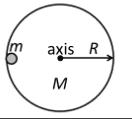# Calculate the mass of the disk?

• alaa amed
The moment of inertia of the disk-clay system about the central axis of the disk is I=1.5kgm2.The mass of the disk is M.The mass of the clay is 0.12kg.The disk's moment of inertia is I=1.5kgm2.The clay's moment of inertia is I=0.12kgm2.The total moment of inertia is I=1.8kgm2.

## Homework Statement

The moment of inertia of the disk-clay system about the central axis of the disk is I = 1.5 kg m2
Disk's mass = M
The clay's mass = 0.12 kg
Calculate the mass of the disk?

I = m r^2

## The Attempt at a Solution

I thought that the moment of inertia may just be the sum of the individual moment of inertia's of the two objects. I know that I for a disk is equal to 1/4 MR^2, but I do not know the one for the clay.[/B]

I thought that the moment of inertia may just be the sum of the individual moment of inertia's of the two objects.
Correct.

I know that I for a disk is equal to 1/4 MR^2, but I do not know the one for the clay.

You sure you've interpreted the axis correctly?
https://en.wikipedia.org/wiki/List_of_moments_of_inertia

Was there a diagram? As it is, we don't know r disk or rclay so can't find mclay.

billy_joule said:
Correct.

You sure you've interpreted the axis correctly?
https://en.wikipedia.org/wiki/List_of_moments_of_inertia

Was there a diagram? As it is, we don't know r disk or rclay so can't find mclay.
Yes. The diagram showed a piece of clay is sitting at the rim of the disk and not sliding on the disk.

Post the diagram and the entire question statement word for word, without it we're just guessing/relying on your interpretation.A horizontal uniform disk with a mass M and a radius R=1.5m is free to rotate about its central axis perpendicular to the disk’s flat surface. A piece of clay with a mass m=0.12kg is sitting at the rim of the disk and does not slide on the disk. The moment of inertia of the disk-clay system about the central axis of the disk is I=1.5kgm2.At time t=0s, a constant external force, F, is applied tangentially at the rim of the disk causing the disk-clay system, initially at rest, to start rotating with a constant angular acceleration ofα=0.25rad/s2.

There's doesn't appear to be a question?
Can you follow the link in post #2 and identify the relevant MOI equations for the disk and clay?

alaa amed said:
perpendicular to the disk’s flat surface
Note that part of the axis specification.